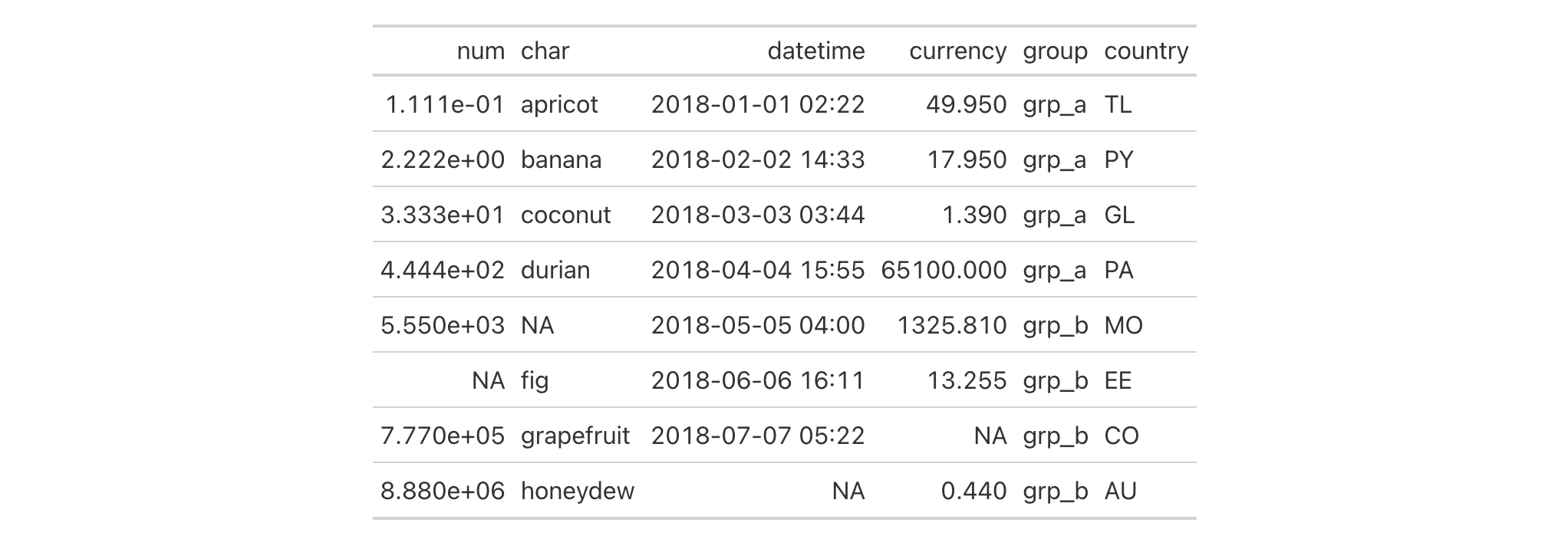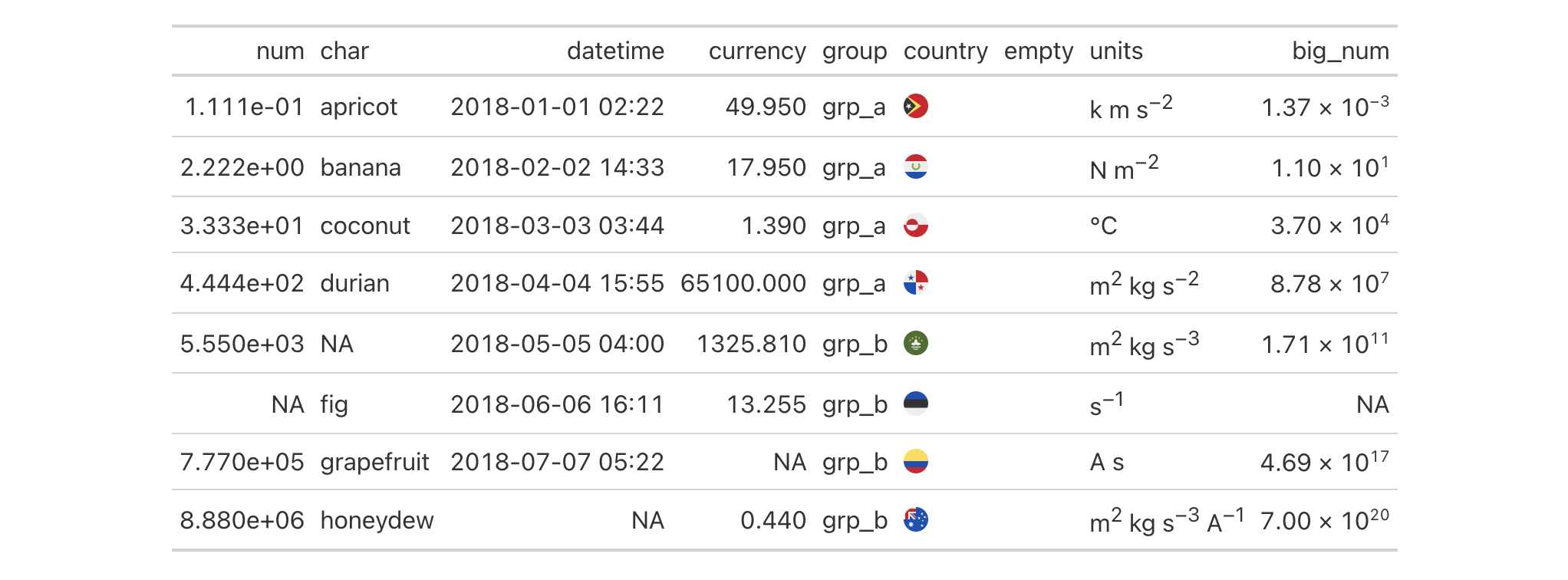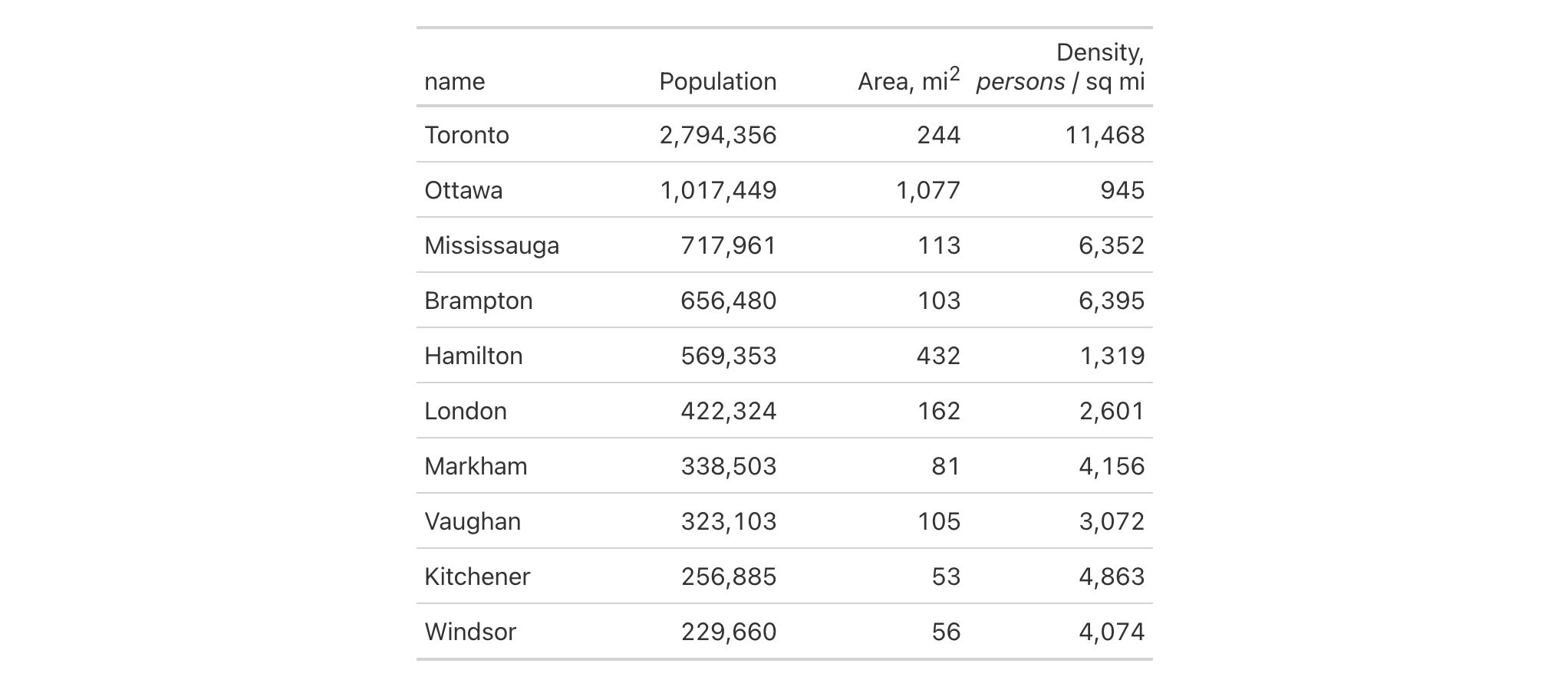We can add new columns to a table with the cols_add() function and it works quite a bit like the dplyr mutate() function. The idea is that you supply name-value pairs where the name is the new column name and the value part describes the data that will go into the column. The latter can: (1) be a vector where the length of the number of rows in the data table, (2) be a single value (which will be repeated all the way down), or (3) involve other columns in the table (as they represent vectors of the correct length). The new columns are added to the end of the column series by default but can instead be added internally by using either the .before or .after arguments. If entirely empty (i.e., all NA) columns need to be added, you can use any of the NA types (e.g., NA, NA_character_, NA_real_, etc.) for such columns.

## Usage

cols_add(.data, ..., .before = NULL, .after = NULL)

## Arguments

.data

The gt table data object

obj:<gt_tbl> // required

This is the gt table object that is commonly created through use of the gt() function.

...

Cell data assignments

<multiple expressions> // (or, use .list)

Expressions for the assignment of cell values to the new columns. Name-value pairs, in the form of <column> = <value vector> will work, so long as any <column> value does not already exist in the table. The <value vector> may be an expression that uses one or more column names in the table to generate a vector of values. Single values in <value vector> will be repeated down the new column. A vector where the length is exactly the number of rows in the table can also be used.

.before, .after

Column used as anchor

<column-targeting expression> // default: NULL (optional)

A single column-resolving expression or column index can be given to either .before or .after. The column specifies where the new columns should be positioned among the existing columns in the input data table. While select helper functions such as starts_with() and ends_with() can be used for column targeting, it's recommended that a single column name or index be used. This is to ensure that exactly one column is provided to either of these arguments (otherwise, the function will be stopped). If nothing is provided for either argument then any new column will be placed at the end of the column series.

## Value

An object of class gt_tbl.

## Targeting the column for insertion with .before or .after

The targeting of a column for insertion is done through the .before or .after arguments (only one of these options should be be used). While tidyselect-style expressions or indices can used to target a column, it's advised that a single column name be used. This is to avoid the possibility of inadvertently resolving multiple columns (since the requirement is for a single column).

## Examples

Let's take a subset of the exibble dataset and make a simple gt table with it (using the row column for labels in the stub). We'll add a single column to the right of all the existing columns and call it country. This new column needs eight values and these will be supplied when using cols_add().

exibble |>
dplyr::select(num, char, datetime, currency, group) |>
gt(rowname_col = "row") |>
country = c("TL", "PY", "GL", "PA", "MO", "EE", "CO", "AU")
)We can add multiple columns with a single use of cols_add(). The columns generated can be formatted and otherwise manipulated just as any column could be in a gt table. The following example extends the first one by adding more columns and immediately using them in various function calls like fmt_flag() and fmt_units().

exibble |>
dplyr::select(num, char, datetime, currency, group) |>
gt(rowname_col = "row") |>
country = c("TL", "PY", "GL", "PA", "MO", "EE", "CO", "AU"),
empty = NA_character_,
units = c(
"k m s^-2", "N m^-2", "degC", "m^2 kg s^-2",
"m^2 kg s^-3", "/s", "A s", "m^2 kg s^-3 A^-1"
),
big_num = num ^ 3
) |>
fmt_flag(columns = country) |>
sub_missing(columns = empty, missing_text = "") |>
fmt_units(columns = units) |>
fmt_scientific(columns = big_num)In this table generated from a portion of the towny dataset, we add two new columns (land_area and density) through a single use of cols_add(). The new land_area column is a conversion of land area from square kilometers to square miles and the density column is calculated by through division of population_2021 by that new land_area column. We hide the now unneeded land_area_km2 with cols_hide() and also perform some column labeling and adjustments to column widths with cols_label() and cols_width().

towny |>
dplyr::select(name, population_2021, land_area_km2) |>
dplyr::filter(population_2021 > 100000) |>
dplyr::arrange(desc(population_2021)) |>
gt() |>
land_area = land_area_km2 / 2.58998811,
density = population_2021 / land_area
) |>
fmt_integer() |>
cols_hide(columns = land_area_km2) |>
cols_label(
population_2021 = "Population",
density = "Density, {{*persons* / sq mi}}",
land_area ~ "Area, {{mi^2}}"
) |>
cols_width(everything() ~ px(120))It's possible to start with an empty table (i.e., no columns and no rows) and add one or more columns to that. You can, for example, use dplyr::tibble() or data.frame() to create a completely empty table. The first cols_add() call for an empty table can have columns of arbitrary length but subsequent uses of cols_add() must adhere to the rule of new columns being the same length as existing.

dplyr::tibble() |>
gt() |>
num = 1:5,
chr = vec_fmt_spelled_num(1:5)
)Tables can contain no rows, yet have columns. In the following example, we'll create a zero-row table with three columns (num, chr, and ext) and perform the same cols_add()-based addition of two columns of data. This is another case where the function allows for arbitrary-length columns (since always adding zero-length columns is impractical). Furthermore, here we can reference columns that already exist (num and chr) and add values to them.

dplyr::tibble(
num = numeric(0),
chr = character(0),
ext = character(0)
) |>
gt() |>
num = 1:5,
chr = vec_fmt_spelled_num(1:5)
)We should note that the ext column did not receive any values from cols_add() but the table was expanded to having five rows nonetheless. So, each cell of ext was by necessity filled with an NA value.

5-7

## Function Introduced

In Development

Other column modification functions: cols_align_decimal(), cols_align(), cols_hide(), cols_label_with(), cols_label(), cols_merge_n_pct(), cols_merge_range(), cols_merge_uncert(), cols_merge(), cols_move_to_end(), cols_move_to_start(), cols_move(), cols_nanoplot(), cols_unhide(), cols_units(), cols_width()